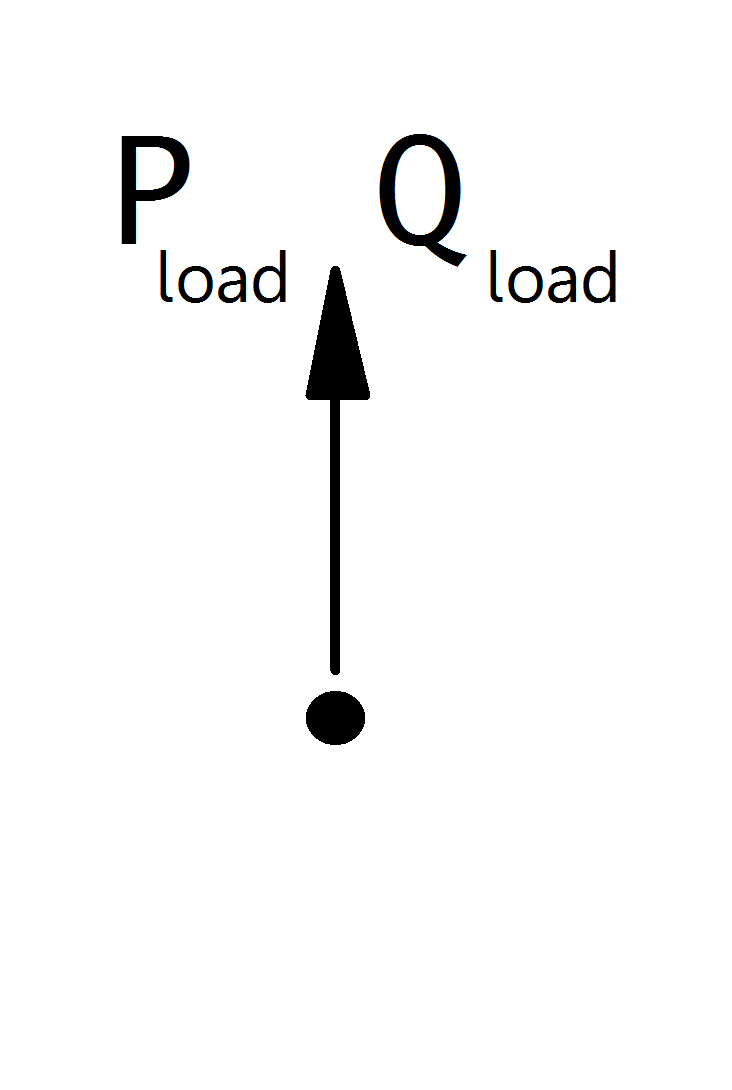# Asymmetric Static Generator¶

Note

Static generators should always have a positive p_mw value, since all power values are given in the generator convention. If you want to model constant power consumption, it is recommended to use a load element instead of a static generator with negative active power value. If you want to model a voltage controlled generator, use the generator element.

## Create Function¶

pandapower.create_asymmetric_sgen(net, bus, p_a_mw=0, p_b_mw=0, p_c_mw=0, q_a_mvar=0, q_b_mvar=0, q_c_mvar=0, sn_mva=nan, name=None, index=None, scaling=1.0, type='wye', in_service=True, k=nan, rx=nan)

Adds one static generator in table net[“asymmetric_sgen”].

Static generators are modelled as negative PQ loads. This element is used to model generators with a constant active and reactive power feed-in. If you want to model a voltage controlled generator, use the generator element instead.

All elements in the grid are modelled in the consumer system, including generators! If you want to model the generation of power, you have to assign a negative active power to the generator. Please pay attention to the correct signing of the reactive power as well.

INPUT:

net - The net within this static generator should be created

bus (int) - The bus id to which the static generator is connected

p_a_mw (float) - The real power of the static generator : Phase A (negative for generation!)

p_b_mw (float) - The real power of the static generator : Phase B(negative for generation!)

p_c_mw (float) - The real power of the static generator : Phase C (negative for generation!)

OPTIONAL:

q_a_mvar (float, default 0) - The reactive power of the sgen : Phase A

q_b_mvar (float, default 0) - The reactive power of the sgen : Phase B

q_c_mvar (float, default 0) - The reactive power of the sgen : Phase C

sn_kva (float, default None) - Nominal power of the sgen

name (string, default None) - The name for this sgen

index (int, None) - Force a specified ID if it is available. If None, the index one higher than the highest already existing index is selected.

scaling (float, 1.) - An OPTIONAL scaling factor to be set customly

type (string, ‘wye’) - Three phase Connection type of the static generator: wye/delta

in_service (boolean) - True for in_service or False for out of service

k (float, NaN) - Ratio of nominal current to short circuit current

rx (float, NaN) - R/X ratio for short circuit impedance. Only relevant if type is specified as motor so that sgen is treated as asynchronous motor

OUTPUT:
index (int) - The unique ID of the created sgen
EXAMPLE:
create_asymmetric_sgen(net, 1, p_b_mw = -120)

## Input Parameters¶

net.asymmetric_sgen

 Parameter Datatype Value Range Explanation name string name of the static generator type string naming conventions: “PV” - photovoltaic system “WP” - wind power system “CHP” - combined heating and power system type of generator bus* integer index of connected bus p_a_mw* float $$\leq$$ 0 active power of the static generator : Phase A[MW] q_a_mvar* float reactive power of the static generator : Phase A [MVar] p_b_mw* float $$\leq$$ 0 active power of the static generator : Phase B[MW] q_b_mvar* float reactive power of the static generator : Phase B [MVar] p_c_mw* float $$\leq$$ 0 active power of the static generator : Phase C[MW] q_c_mvar* float reactive power of the static generator : Phase C [MVar] sn_mva float $$>$$ 0 rated power ot the static generator [MVA] scaling* float $$\geq$$ 0 scaling factor for the active and reactive power max_p_mw** float Maximum active power [MW] min_p_mw** float Minimum active power [MW] max_q_mvar** float Maximum reactive power [MVar] min_q_mvar** float Minimum reactive power [MVar] controllable** bool States if sgen is controllable or not, sgen will not be used as a flexibilty if it is not controllable k*** float $$\geq$$ 0 Ratio of nominal current to short circuit current rx*** float $$\geq$$ 0 R/X ratio for short circuit impedance. Only relevant if type is specified as motor so that sgen is treated as asynchronous motor in_service* boolean True / False specifies if the generator is in service.

*necessary for executing a power flow calculation
**optimal power flow parameter

## Electric Model¶

Static Generators are modelled as PQ-buses in the power flow calculation:The PQ-Values are calculated from the parameter table values as:

\begin{align*} P_{sgen} &= p\_mw \cdot scaling \\ Q_{sgen} &= q\_mvar \cdot scaling \\ \end{align*}

Note

The apparent power value sn_mva is provided as additional information for usage in controller or other applications based on panadapower. It is not considered in the power flow!

## Result Parameters¶

net.asymmetric_sgen

 Parameter Datatype Explanation p_a_mw float resulting active power demand after scaling : Phase A [MW] q_a_mvar float resulting reactive power demand after scaling : Phase A [MVar] p_b_mw float resulting active power demand after scaling : Phase B [MW] q_b_mvar float resulting reactive power demand after scaling : Phase B [MVar] p_c_mw float resulting active power demand after scaling : Phase C [MW] q_c_mvar float resulting reactive power demand after scaling : Phase C [MVar]

The power values in the net.res_sgen table are equivalent to $$P_{sgen}$$ and $$Q_{sgen}$$.